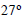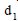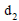NEET  >  Test: Physics - 10

# Test: Physics - 10

Test Description

## 45 Questions MCQ Test NEET Mock Test Series | Test: Physics - 10

Test: Physics - 10 for NEET 2023 is part of NEET Mock Test Series preparation. The Test: Physics - 10 questions and answers have been prepared according to the NEET exam syllabus.The Test: Physics - 10 MCQs are made for NEET 2023 Exam. Find important definitions, questions, notes, meanings, examples, exercises, MCQs and online tests for Test: Physics - 10 below.
Solutions of Test: Physics - 10 questions in English are available as part of our NEET Mock Test Series for NEET & Test: Physics - 10 solutions in Hindi for NEET Mock Test Series course. Download more important topics, notes, lectures and mock test series for NEET Exam by signing up for free. Attempt Test: Physics - 10 | 45 questions in 45 minutes | Mock test for NEET preparation | Free important questions MCQ to study NEET Mock Test Series for NEET Exam | Download free PDF with solutions
 1 Crore+ students have signed up on EduRev. Have you?
Test: Physics - 10 - Question 1

### For Boyle’s law to hold good, the gas should be

Test: Physics - 10 - Question 2

### A bulb contains 1 mole of hydrogen and 1 mole of oxygen at temperature T. The ratio of rms values of velocity of hydrogen molecules to that of oxygen molecule is

Test: Physics - 10 - Question 3

### An ideal gas is heated from 270  to 6270C  at constant pressure. If initial volume was 4m3then the final volume of gas will be

Test: Physics - 10 - Question 4

When we heat a gas sample fromto 3270C then the initial average kinetic energy of the molecules was E. What will be the average kinetic energy after heating?

Test: Physics - 10 - Question 5
If at the same temperature and pressure,the densities of two diatomic gases areandrespectively,then the ratio of velocities of sound in these gases is
Test: Physics - 10 - Question 6

A molecule of mass m of an ideal gas collides with the wall of the vessel with the same velocity. The change in the linear momentum of the molecule will be

Test: Physics - 10 - Question 7
Rate of diffusion is
Test: Physics - 10 - Question 8
The instrument used to measure the temperature of the source from its thermal radiation is
Test: Physics - 10 - Question 9

A Centigrade and Fahrenheit thermometers are dipped in boling water. The Fehrenheit thermometer registers a temperature of 1400.The temperature as registered by the centigrade thermometer is

Test: Physics - 10 - Question 10
Which one of the following is not a thermodynamical co-ordinate ?
Test: Physics - 10 - Question 11

The mean kinetic energy of a molecule of a gas at 300 K is 6.21 x 10-21J  The root mean square velocity of a molecule of Hgas at this temperature will be (R = 8.3 J/mole K, N = 6.02 x 1023 / mole mass of the hydrogen moleucle = 2 x 1.67 x 10-27 kg

Test: Physics - 10 - Question 12

At what temperautre will the rms velocity of oxygen moleucle be sufficient, so as to escape from the earth? Escape velocity from the earth is 11.0 km/s and the mass of 1 moleucle of oxygen 5.34 x 10-26 (Boltzman constant k = 1.38 x 10-23 J/K)

Test: Physics - 10 - Question 13

A steel rod 25 cm lo (Y = 20 x 1011 dyne/cm2)ng has a cross-sectional area of 0.8 cm2. What would be the force required to strech this rod by the same amount as the expansion produced by heating it through 100C ? (α = 10-5/0C

Test: Physics - 10 - Question 14

A steel scale measures the lenght of a copper wire as 80.0 cm, when both are at 200C the calibration temperature for the scale. What would the scale read for the lenght of the rod when both are at 400  (Given: α for steel = 11 x 10-6/0C and α for Cu = 17 x 10-6/0C)

Test: Physics - 10 - Question 15

A sample of gas expands from volume V1 to V2. The amount of work done by the gas is greatest, when the expansion is

Test: Physics - 10 - Question 16
During the adiabatic expansion of 2 moles of a gas the internal energy of a gas is found to decrease by 2J. The work done during the process on gas will be equal to
Test: Physics - 10 - Question 17

In an adiabatic change, the pressure and temperature of a monoatomic gas are related with relation as P α TC where C is equal to

Test: Physics - 10 - Question 18

Two samples of air X and Y having same composition and initially at the same temperature and pressure are compressed from volume V to V/2. X is compressed isothermally and Y adiabatically. Then, the final pressure will be

Test: Physics - 10 - Question 19
Magnitude of slope of an adiabatic curve is always
Test: Physics - 10 - Question 20

Air is filled in a bottle at atmoshperic pressure and it is cordked at 350C . If the cork can come out at 3 atmospheric pressure then up to what temperature should the bottle be heated in order to remove the cork?

Detailed Solution for Test: Physics - 10 - Question 20

Solution :- P1 V1 / T1 = P2 V2 / T2

Volume is constant (bottle volume). So,

P1 / T1 = P2 / T2

1 / (273+35) = 3 / T2

So temperature to be heated is, 651 deg C.

Test: Physics - 10 - Question 21

A perfect gas goes from state A to another state B by absorbing 8 x 105J of heat and doing 6.5 x 105J external work. It is now transferred between the same two states in another process in which it absorbs 105J of heat. Then, in the second process

Test: Physics - 10 - Question 22
If a system undergoes contraction of volume, then the work done by the system will be
Test: Physics - 10 - Question 23

First law of thermodynamics is a special case of

Test: Physics - 10 - Question 24

An ideal refrigerator has a freezer at a temperature of -130CThe coefficient of performance of the engine is 5. The temperature of the air (to which heat is rejected) will be

Test: Physics - 10 - Question 25
A Carnot reversible engine converts 1/6 of heat input work. When the temperature of the sink is reduced by 62 K, the efficiency of Carnot’s cycle becomes1/3. The temperature of the source and sink will be
Test: Physics - 10 - Question 26

Compressional wave pules are sent to the bottom of sea from a ship and echo is heard after 2s. If bulk modulus of elasticity of water is 2 x 109N/m2 and density of water at 40C is 1 x 103kg/m3 The depth of the sea will be

Test: Physics - 10 - Question 27

A quantity of heat required to change the unit mass of a solid substance to its liquid state, while the temperature remains constant, is known as

Test: Physics - 10 - Question 28
When a solid is converted into a gas,directly by heating then this process is known as
Test: Physics - 10 - Question 29
The latent heat of vapourization of water is 2240 J.If the work done in the process of vapourization of 1 g is 168 J, then increase in internal energy will be
Test: Physics - 10 - Question 30

If the temperature of a black body increases from 70C to 2870C then, the rate of energy of radiation is

Test: Physics - 10 - Question 31
When the thermal conductivity of a rod is 2. What is its thermal resistivity ?
Test: Physics - 10 - Question 32

On a cold morning, a metal surface on touching is felt colder than a wooden surface, because the metal has

Test: Physics - 10 - Question 33
Heat travels through vaccum by
Test: Physics - 10 - Question 34
The sum emits a light with maximum wavelength 510 nm while another star emits a light with maximum wavelength of 350 nm. The ratio of surface temperature of sun and the star will be
Test: Physics - 10 - Question 35

A black body radiates heat energy at the rate of 2 x 105J/s-m2  at 400 K. The temperature of the black body at which rate of heat radiation is 32 x 105 J/s-m2 will be

Test: Physics - 10 - Question 36

A star will appear blue, if the wavelength of maximum emission is in the range 450 to 490 nm. The range of temperature corresponding to which a star will appear blue, will be (Wien’s constant b = 2.897 x 10-3mK)

Test: Physics - 10 - Question 37

Direction : Read the following question and choose
A. If both Assetion and Reason are correct and reason is the correct exaplanation of assertion
B. If both Assertion and Reason are true, but Reason is not correct explanation of the Assertion
C. If Assertion is true, but the Reason is false
D. If Assertion is false, but the Reason is true
E. If both assertion and reason are false
Assertion : A body that is a good ratiator is also a good absorber of radiation at a given wavelength
Reason : According to Kirchhoff ’s law the absorptivity of a body is equal to its emissivity at a given wavelength

Test: Physics - 10 - Question 38
Direction : Read the following question and choose
A. If both Assetion and Reason are correct and reason is the correct exaplanation of assertion
B. If both Assertion and Reason are true, but Reason is not correct explanation of the Assertion
C. If Assertion is true, but the Reason is false
D. If Assertion is false, but the Reason is true
E. If both assertion and reason are false
Assertion : Temperatures near the sea coast are moderate.
Reason : Water has a high thermal conductivity
Test: Physics - 10 - Question 39
Direction : Read the following question and choose
A. If both Assetion and Reason are correct and reason is the correct exaplanation of assertion
B. If both Assertion and Reason are true, but Reason is not correct explanation of the Assertion
C. If Assertion is true, but the Reason is false
D. If Assertion is false, but the Reason is true
E. If both assertion and reason are false
Assertion : It is hotter over the top of a fire than at the same distance on the sides
Reason : Air surrounding the fire conducts more heat upward
Test: Physics - 10 - Question 40
Direction : Read the following question and choose
A. If both Assetion and Reason are correct and reason is the correct exaplanation of assertion
B. If both Assertion and Reason are true, but Reason is not correct explanation of the Assertion
C. If Assertion is true, but the Reason is false
D. If Assertion is false, but the Reason is true
E. If both assertion and reason are false
Assertion : Air quickly leaking out of a balloon becomes coolers
Reason : The leaking air undergoes adiabatic expansion
Test: Physics - 10 - Question 41
Direction : Read the following question and choose
A. If both Assetion and Reason are correct and reason is the correct exaplanation of assertion
B. If both Assertion and Reason are true, but Reason is not correct explanation of the Assertion
C. If Assertion is true, but the Reason is false
D. If Assertion is false, but the Reason is true
E. If both assertion and reason are false
Assertion : Work and heat are two equivalent form of energy
Reason : Work is the transfer of mechanical energy irrespective of temperature difference, whereas heat is the transfer of thermal energy beacuse of temperature difference only
Test: Physics - 10 - Question 42
Direction : Read the following question and choose
A. If both Assetion and Reason are correct and reason is the correct exaplanation of assertion
B. If both Assertion and Reason are true, but Reason is not correct explanation of the Assertion
C. If Assertion is true, but the Reason is false
D. If Assertion is false, but the Reason is true
E. If both assertion and reason are false
Assertion : Specific heat of a body is always greater than its thermal capacity
Reason : Thermal capacity is the heat required for raising temperature of unit mass of the body through unit degree
Test: Physics - 10 - Question 43
Direction : Read the following question and choose
A. If both Assetion and Reason are correct and reason is the correct explanation of assertion
B. If both Assertion and Reason are true, but Reason is not correct explanation of the Assertion
C. If Assertion is true, but the Reason is false
D. If Assertion is false, but the Reason is true
E. If both assertion and reason are false
Assertion : The molecules at 0oC ice and water will have same potential energy
Reason : Potential energy depends only on temperature of the system
Test: Physics - 10 - Question 44
Direction : Read the following question and choose
A. If both Assertion and Reason are correct and reason is the correct explanation of assertion
B. If both Assertion and Reason are true, but Reason is not correct explanation of the Assertion
C. If Assertion is true, but the Reason is false
D. If Assertion is false, but the Reason is true
E. If both assertion and reason are false
Assertion : In the upper part of the atmosphere, the temperature of air is of the order of 1000 K, even than it is quite cold there
Reason : Molecule density at high altitudes is low
Test: Physics - 10 - Question 45

Direction : Read the following question and choose
A. If both Assertion and Reason are correct and reason is the correct explanation of assertion
B. If both Assertion and Reason are true, but Reason is not correct explanation of the Assertion
C. If Assertion is true, but the Reason is false
D. If Assertion is false, but the Reason is true
E. If both assertion and reason are false
Assertion : The ratio of specific heat of gas at constant pressure and specific heat at constant volume is more for helium gas, than for hydrogen gas
Reason : Atomic mass of helium is more than that of hydrogen

## NEET Mock Test Series

1 videos|25 docs|144 tests
 Use Code STAYHOME200 and get INR 200 additional OFF Use Coupon Code
Information about Test: Physics - 10 Page
In this test you can find the Exam questions for Test: Physics - 10 solved & explained in the simplest way possible. Besides giving Questions and answers for Test: Physics - 10, EduRev gives you an ample number of Online tests for practice

## NEET Mock Test Series

1 videos|25 docs|144 tests

### How to Prepare for NEET

Read our guide to prepare for NEET which is created by Toppers & the best Teachers